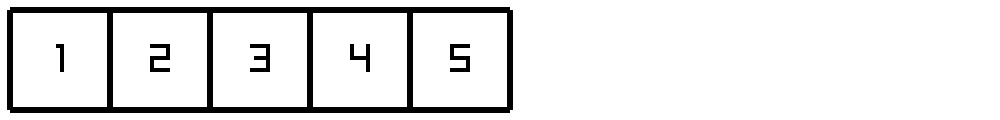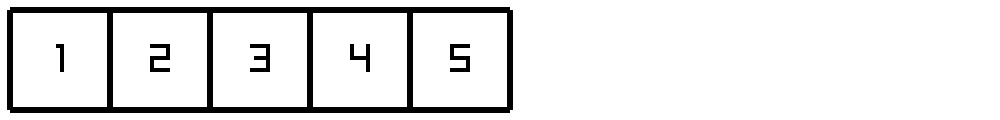# Lesson 9 - More on functions## Introduction

For this lesson, we will take a closer look at how functions work, and learn about how data is stored and used.

In D, all code is encapsulated into a function. But what is a function? A function is a list of sequential statements that represent very closely what the computer should do when you call the function. It has a definite beginning, and a definite end, but the flow of control can choose different paths (or branches), and even loop back to previous points.

What about the variables that are declared in the function? How does the computer/compiler keep track of these things? How do returns work? Let's look at some of these questions, and also investigate how to affect things outisde the function.

## The Stack Frame

A program running inside a computer has what is called Random Access Memory or RAM to use for storing information. When the compiler reads that you want to store some data in a variable, it tells the computer that it will be using some of that RAM to store that variable. The compiler takes note of all the variables you need to store and builds a stack frame or a segment of data that it will need to execute the function. A stack frame has 2 parts, the parameters for the function, and the variables you declared to use inside the function.

For example, let's say we have the function `int addall(int a, int b)`, and we called it like `addall(1, 5)`. The stack frame will look something like this:

1
5

This is what the stack frame looks like just before the `addall` function starts running. This stack frame is set up by the call from the calling function.

Let's say the `addall` function adds all the numbers from `a` to `b`. It might be implemented like this:

``````int addall(int a, int b) {
int r = 0;
foreach(i; a .. b)
{
r += i;
}
return r;
}``````

This adds to the stack frame, which the compiler sets up just after entering the function:

1
5
0
33471623

That number for `i` is not a typo! Before it is used, the memory that is used for `i` has not been set, and whatever is in that memory cell is what is shown. But when it gets to the `foreach` loop, `i` will be set to 1 for the first iteration of the loop.

If you used a debugger on this code, you might see more variables, which the compiler generates to run the loop. Those would also go into the stack frame.

Once the loop is done, the `return` statement stuffs the value into another piece of memory to give back to the calling function, where it is waiting for the result. Then the stack frame is removed, and that memory is ready to be used for another function.

What if `addall` called more functions? Those would then be added onto the stack with a frame for each of the functions it called. A stack is just like a stack of books or dishes. You put things on top of it, and then you take them off of the top. So in this way, you can have memory to store the stack frame for any function you call, using the RAM of the computer.

## Recursion

An interesting thing to realize is that every call to a function has its own stack frame assigned to it. But what if a function calls itself? What happens is just like any other function call, a new stack frame is added, and the new parameters and variables, and return values all go onto that new frame.

This concept is called recursion. Let's rewrite the `addall` function such that it uses recursion.

``````int addall(int a, int b) {
if(a == b) {
return 0;
}
else {
return a + addall(a + 1, b);
}
}``````

Notice the first thing that we do is check for the stopping condition. Since we don't have a loop, we need some way to stop. If we never stopped, the code would recurse until the computer runs out of memory (and it would crash the program). Our stopping condition is when `a == b`. When this occurs, it would be the same as the end of the loop (when `i == b` in the `foreach` loop).

If that is true, we return the starting sum, or 0. This is equivalent to the loop version setting `r = 0`.

If that is false, we need to add more things. So first we add the "rest" of the values together, from `a + 1` to `b`, and then we can add `a` to it as well. and we just return the value.

Those of you familiar with mathematical notation will find this very familiar for defining a mathematical function. In fact, many programming languages require this kind of recursive definition.

So let's say we call the function `addall(1, 5)`. Inside the function, 1 is not equal to 5, so `addall(2, 5)` is added to the stack. Inside that function, `addall(3, 5)` is called, and so on. At the end, inside `addall(5, 5)`, the call stack looks like this:

1
5
2
5
3
5
4
5
5
5

The last stack frame returns 0. Then the next stack frame returns 4 (4 + 0 == 4). The next stack frame receives that 4 and adds it to 3 making 7. The next stack frame returns 9, and the final stack frame returns 10. Then, whichever function called the original `addall` function gets 10 and uses that for its purposes.

## Reference parameters

You may notice that every instance of the `addall` function has its own copy of `a`, and `b`. However, this means you are copying the value onto the stack. What if you wanted to affect the original variable directly? There is actually a way to do this, and it's called passing by reference. In D, the way to tell the compiler that you want to pass by reference is to use the keyword `ref` on the parameter. This goes before the type in the parameter definition:

``````void doubleit(ref int x) {
x *= 2;
}``````

Notice that the function does not return anything, because it doesn't have to. `x` is changed in place. If we did not have the `ref` attribute, then `x` would be a copy of the parameter, and you would double it. When the stack frame went away, that copy would be gone, and nobody would ever know your function did anything!

But since we passed by reference, the caller will see the change in the original variable it passed in for `x`. Because there needs to be a place to refer to in memory, you are not allowed to pass expressions to the function, such as `5` or `a + 2`. It must be an actual variable.

How this works internally is that there is still a space reserved on the stack for `x`. But instead of storing a copy, it stores a pointer to the data in memory. It can do this because every piece of memory has an address, just like your house has an address. If you know the address, you can change the value. It's not important right now to know how addresses work, but it is useful to have an understanding of how references work.

## Code to remove an array value

Let's go through somewhat useful code to remove an element from an array. An array is kind of like a stack, in that you can only add to the end and remove from the end. What if you want to remove a value from the middle of the array?

In order to do that, we must replace the element being removed with another element. There are two ways to do this, and we will look at both.

### Stable remove

The first mechanism is a stable removal of the element. Stable means that the rest of the elements keep their same ordering. Stability is important if you care about the order of elements in the array.

In order for the array elements to keep the same order, we need to copy things over one element. This means each element of the array needs to move one element to the left. This is illustrated via the following animation of removing the middle element `3` from the array `[1, 2, 3, 4, 5]`:To implement this, we need to loop over the array elements. Given any array element index, we know it needs to copy from the next index. Therefore, we can implement it like this:

``````int elementToRemove = 2; // remember, 2 is the *third* element, they start at 0
foreach(i; elementToRemove .. arr.length - 1) {
arr[i] = arr[i + 1]; // copy each element down
}``````

At the end, this leaves us with the last element still there: `[1, 2, 4, 5, 5]`. For the last step, we need to remove the last element. We can add or remove elements from an array by setting the length to a new value:

``arr.length -= 1;``

Now the array is just `[1, 2, 4, 5]`, as we desired! Because we did a stable remove, the elements are all still in the same order as they were in the original array.

### Non-stable remove

There is another way to remove an element, which does not keep the order stable. This way does not involve any loop! Since we can only remove the last element, the goal is to make the last element unused. To do that, the original last element (`5`) has to go somewhere. The perfect place is the spot we are removing. So we can just move the last element into that spot, and then we are done. Of course we still have to subtract one from the length. The following animation shows how this occurs:The following code is one way to remove the element:

``````int elementToRemove = 2;
arr[elementToRemove] = arr[arr.length - 1];
arr.length -= 1;``````

At the end of the code, removing the `3` from `[1, 2, 3, 4, 5]` results in `[1, 2, 5, 4]`.